# High School Physics : Calculating Total Resistance

## Example Questions

2 Next →

### Example Question #31 : Electricity And Magnetism

What is the total resistance of a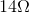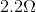, and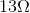series circuit?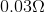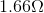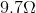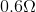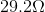Explanation:

The formula for resistors in series is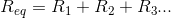.

Plug in our given values.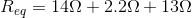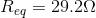### Example Question #1 : Electricity And Magnetism

There are three resistors in parallel in a circuit with resistances of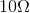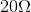, and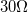.

What is the equivalent resistance?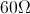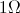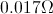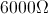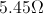Explanation:

The equation for resistors in parallel is: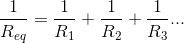We are given the values of the resistors. Using this formula, we can solve for the equivalent resistance.

Plug in the given values and solve.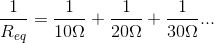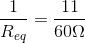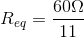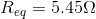2 Next →

### All High School Physics Resources